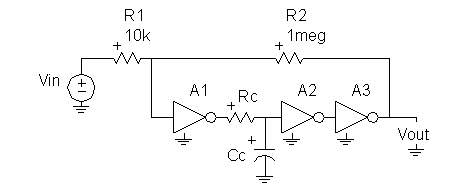# Would somebody help me about how to find the dominant pole?

Status
Not open for further replies.

#### kyliv

##### Newbie level 3Three inverters are cascaded to create an OPAMP, which, in turn is configured as an inverting amplifier. Assuming all three inverters are identical with a gain a0 = -100 and a single pole at 100 kHz, calculate the dominant pole frequency needed to get 45° of phase margin in the overall inverting OPAMP topology. (this pole will be set by the Rc and Cc network.) overall inverting OPAMP topology.#### gingerjiang

##### Full Member level 3Re: Would somebody help me about how to find the dominant po

you can model the inverter I(s)=-a0/(1+s/w), then calculate the transfer function of opamp to determinate the pole.

### kyliv

Points: 2

#### kyliv

##### Newbie level 3Re: Would somebody help me about how to find the dominant po

Thanks! I use A(S)=-a0/[(1-s/p1)+a0f) and find the solution.

Status
Not open for further replies.